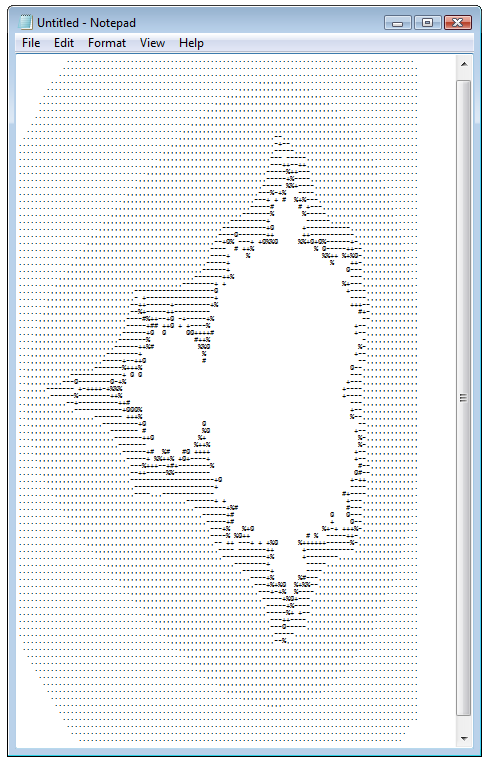The bar for entry into CodeSOD is pretty straight forward: professionally-developed code that elicits that certain What The— reaction. Though there have been a few exceptions over the years, generally speaking, student code, hobbyist code, and amateur code need not apply. That said, I'd like to try something a little different today. Today's example is not technically professionally-developed, it's a Stupid Coding Trick.

"So I was bored at work one day," Graeme Job explains, "and wondered, what's the most useless thing I could do with my time without actually doing anything. Then it hit me. I could use T-SQL to generate... Mandelbrot."

Graeme continued, "Following is a single T-SQL SELECT statement that generates a text-representation of a Mandelbrot Set. The results are best viewed in text-mode."

-- AUTHOR: GRAEME JOB
-- CREATED: 12-OCT-2008
-- BECAUSE: SINGLE SQL COMMAND < 50 LINES. JUST BECAUSE.
WITH
XGEN(X, IX) AS (              -- X DIM GENERATOR
SELECT CAST(-2.2 AS FLOAT) AS X, 0 AS IX UNION ALL
SELECT CAST(X + 0.031 AS FLOAT) AS X, IX + 1 AS IX
FROM XGEN
WHERE IX < 100
),
YGEN(Y, IY) AS (              -- Y DIM GENERATOR
SELECT CAST(-1.5 AS FLOAT) AS Y, 0 AS IY UNION ALL
SELECT CAST(Y + 0.031 AS FLOAT) AS Y, IY + 1 AS IY
FROM YGEN
WHERE IY < 100
),
Z(IX, IY, CX, CY, X, Y, I) AS (           -- Z POINT ITERATOR
SELECT IX, IY, X, Y, X, Y, 0
FROM XGEN, YGEN
UNION ALL
SELECT IX, IY, CX, CY, X * X - Y * Y + CX AS X, Y * X * 2 + CY, I + 1
FROM Z
WHERE X * X + Y * Y < 16
AND I < 100
)
SELECT REPLACE(REPLACE(REPLACE(REPLACE(REPLACE(REPLACE(REPLACE(REPLACE(REPLACE(REPLACE(
REPLACE(REPLACE(REPLACE(REPLACE(REPLACE(REPLACE(REPLACE(REPLACE(REPLACE(REPLACE(
REPLACE(REPLACE(REPLACE(REPLACE(REPLACE(REPLACE(
(X0+X1+X2+X3+X4+X5+X6+X7+X8+X9+X10+X11+X12+X13+X14+X15+X16+X17+X18+X19+
X20+X21+X22+X23+X24+X25+X26+X27+X28+X29+X30+X31+X32+X33+X34+X35+X36+X37+X38+X39+
X40+X41+X42+X43+X44+X45+X46+X47+X48+X49+X50+X51+X52+X53+X54+X55+X56+X57+X58+X59+
X60+X61+X62+X63+X64+X65+X66+X67+X68+X69+X70+X71+X72+X73+X74+X75+X76+X77+X78+X79+
X80+X81+X82+X83+X84+X85+X86+X87+X88+X89+X90+X91+X92+X93+X94+X95+X96+X97+X98+X99),
'A',' '),   'B','.'),   'C',','),   'D',','),   'E',','),   'F','-'),   'G','-'),
'H','-'),   'I','-'),   'J','-'),   'K','+'),   'L','+'),   'M','+'),   'N','+'),
'O','%'),   'P','%'),   'Q','%'),   'R','%'),   'S','@'),   'T','@'),   'U','@'),
'V','@'),   'W','#'),   'X','#'),   'Y','#'),   'Z',' ')
FROM (
SELECT 'X' + CAST(IX AS VARCHAR) AS IX,
IY,   SUBSTRING('ABCDEFGHIJKLMNOPQRSTUVWXYZ', ISNULL(NULLIF(I, 0), 1), 1) AS I
FROM Z) ZT
PIVOT (
MAX(I) FOR IX IN (
X0,X1,X2,X3,X4,X5,X6,X7,X8,X9,X10,X11,X12,X13,X14,X15,X16,X17,X18,X19,
X20,X21,X22,X23,X24,X25,X26,X27,X28,X29,X30,X31,X32,X33,X34,X35,X36,X37,X38,X39,
X40,X41,X42,X43,X44,X45,X46,X47,X48,X49,X50,X51,X52,X53,X54,X55,X56,X57,X58,X59,
X60,X61,X62,X63,X64,X65,X66,X67,X68,X69,X70,X71,X72,X73,X74,X75,X76,X77,X78,X79,
X80,X81,X82,X83,X84,X85,X86,X87,X88,X89,X90,X91,X92,X93,X94,X95,X96,X97,X98,X99)
) AS PZT

As for the aforementioned results, I've taken the liberty of pasting them into notepad and snapping a screenshot.Have any of your own stupid coding tricks to share? Then send 'em on in. The goal of a stupid coding trick isn't obfuscation per se... just, well, stupid awesomeness. Kinda like a quine, except even more useless.[Advertisement] BuildMaster allows you to create a self-service release management platform that allows different teams to manage their applications. Explore how!